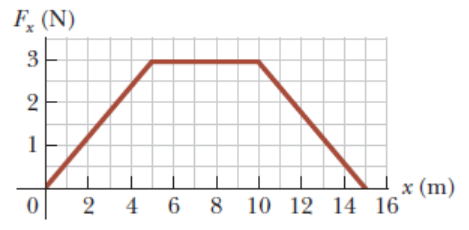# Problem: A particle is subject to a force Fx that varies with position as shown in the following figure. Find the work done by the force on the particle as it moves from x = 0 to x = 2.00 m.

###### FREE Expert Solution

In a Force-position time graph, the work done is equal to the area under the curve.

From x = 0m to x = 2 m, the area is a trangle.

87% (359 ratings)###### Problem Details

A particle is subject to a force Fx that varies with position as shown in the following figure. Find the work done by the force on the particle as it moves from x = 0 to x = 2.00 m.